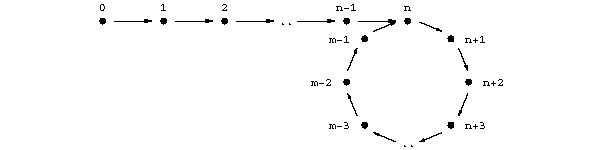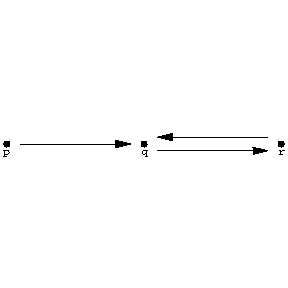# Ockham algebra

(diff) ← Older revision | Latest revision (diff) | Newer revision → (diff)

A bounded distributive lattice $( L; \wedge, \lor,0,1 )$ together with a dual lattice endomorphism $f$, i.e., a mapping $f : L \rightarrow L$ such that the de Morgan laws $f ( x \lor y ) = f ( x ) \wedge f ( y )$ and $f ( x \lor y ) = f ( x ) \wedge f ( y )$ hold for all $x,y \in L$. The class $\mathbf O$ of Ockham algebras is equational (i.e., is a variety; cf. also Algebraic systems, variety of). The Berman class $\mathbf K _ {p,q }$ is the subclass obtained by imposing on the dual endomorphism $f$ the restriction $f ^ {2p+q } = f ^ {q}$( $p \geq 1$, $q \geq 0$). The Berman classes are related as follows:

$$\mathbf K _ {p,q } \subseteq \mathbf K _ {p ^ \prime ,q ^ \prime } \iff p \mid p ^ \prime , q \leq q ^ \prime .$$

The smallest Berman class is therefore the class $\mathbf K _ {1,0 }$ described by the equation $f ^ {2} = { \mathop{\rm id} }$ and is the class $\mathbf M$ of de Morgan algebras. Perhaps the most important Berman class is $\mathbf K _ {1,1 }$, described by $f ^ {3} = f$. This can be characterized as the class of Ockham algebras $L$ such that $( f ( L ) ;f ) \in \mathbf M$. It contains also the class $\mathbf M \mathbf S$ of $MS$- algebras $( { \mathop{\rm id} } \leq f ^ {2} )$, and, in particular, the class of $\mathbf S$ of Stone algebras (add the relation $x \wedge f ( x ) = 0$).

An Ockham algebra congruence is an equivalence relation that has the substitution property for both the lattice operations and the unary operation $f$. A basic congruence is $\Phi _ {n}$, defined by

$$( x,y ) \in \Phi _ {n} \iff f ^ {n} ( x ) = f ^ {n} ( y ) .$$

If $( L;f ) \in \mathbf K _ {p,q }$, then, for $n \leq q$, $L/ \Phi _ {n} \sim f ^ {n} ( L ) \in \mathbf K _ {p,q - n }$, where $\sim$ indicates an isomorphism when $n$ is even and a dual isomorphism when $n$ is odd.

An Ockham algebra $( L;f )$ is subdirectly irreducible if it has a smallest non-trivial congruence. Every Berman class contains only finitely many subdirectly irreducible algebras, each of which is finite.

The class $\mathbf K _ \omega$ of $\mathbf O$ is given by

$$( L;f ) \in \mathbf K _ \omega \iff ( \forall x ) ( \exists m \neq 0,n ) f ^ {m + n } ( x ) = f ^ {n} ( x ) ;$$

it is a locally finite generalized variety that contains all of the Berman classes. If $( L;f ) \in \mathbf K _ \omega$, then $L$ is subdirectly irreducible if and only if the lattice of congruences of $L$ reduces to the chain

$$\omega = \Phi _ {0} \prec \Phi _ {1} \prec \dots \prec \Phi _ \omega \prec \iota$$

where $\Phi _ \omega = \cup _ {i \geq 0 } \Phi _ {i}$. If $L \in \mathbf K _ {p,q }$, then $\Phi _ \omega = \Phi _ {q}$.

Ockham algebras can also be obtained by topological duality. Recall that a set $D$ in a partially ordered set $P$ is called a down-set if $a \leq b$, $b \in D$, implies $a \in D$. Dually, $U \subset P$ is called an up-set if $a \leq b$, $b \in U$, implies $b \in U$. An ordered topological space $( X; \tau, \leq )$( cf. also Order (on a set)) is said to be totally order-disconnected if, whenever $x \Nle y$, there exists a closed-and-open down-set $U$ such that $y \in U$ and $x \notin U$. A Priestley space is a compact totally order-disconnected space. An Ockham space is a Priestley space endowed with a continuous order-reversing mapping $g$. The important connection with Ockham algebras was established by A. Urquhart and is as follows. If $( X;g )$ is an Ockham space and if ${\mathcal O} ( X )$ denotes the family of closed-and-open down-sets of $X$, then $( {\mathcal O} ( X ) ;f )$ is an Ockham algebra, where $f$ is given by $f ( A ) = X \setminus g ^ {- 1 } ( A )$. Conversely, if $( L;f )$ is an Ockham algebra and if $I _ {p} ( L )$ denotes the set of prime ideals of $L$, then, if $I _ {p} ( L )$ is equipped with the topology $\tau$ which has as base the sets $\{ {x \in I _ {p} ( L ) } : {x \ni a } \}$ and $\{ {x \in I _ {p} ( L ) } : {x \Nso a } \}$ for every $a \in L$, $( I _ {p} ( L ) ;g )$ is an Ockham space, where $g ( x ) = \{ {a \in L } : {f ( a ) \notin x } \}$. Moreover, these constructions give a dual categorical equivalence. In the finite case the topology "evaporates" ; the dual space of a finite Ockham algebra $L$ consists of the ordered set $I$ of join-irreducible elements together with the order-reversing mapping $g$.

Duality produces further classes of Ockham algebras. For $m > n \geq 0$, let $\mathbf P _ {m,n }$ be the subclass of $\mathbf O$ formed by the algebras whose dual space satisfies $g ^ {m} = g ^ {n}$. Then every Berman class is a $\mathbf P _ {m,n }$; more precisely, $\mathbf K _ {p,q } = \mathbf P _ {2p + q,q }$. If $( X;g )$ is the dual space of $( L;f )$, let, for every $x \in X$, $g ^ \omega \{ x \} = \{ {g ^ {n} ( x ) } : {n \in \mathbf N } \}$. If $( L;f ) \in \mathbf O$ is finite, then $( L;f )$ is subdirectly irreducible if and only if there exists an $x \in X$ such that $g ^ \omega \{ x \} = X$. The dual space of a subdirectly irreducible Ockham algebra in $\mathbf P _ {m,n }$ can therefore be represented as follows (here the order is ignored and the arrows indicate the action of $g$):Figure: o110030a

The subdirectly irreducible Ockham algebra that corresponds to this discretely ordered space is denoted by $L _ {m,n }$. In particular, $( L _ {3,1 } ;f )$ is the algebra whose dual space isFigure: o110030b

and is described as follows:Figure: o110030c

The subdirectly irreducible algebras in $\mathbf K _ {1,1 } = \mathbf P _ {3,1 }$ are the nineteen subalgebras of $( L _ {3,1 } ; f )$. Using a standard theorem of B.A. Davey from universal algebra, it is possible to describe completely the lattice of subvarieties of $\mathbf K _ {1,1 }$.

How to Cite This Entry:
Ockham algebra. Encyclopedia of Mathematics. URL: http://encyclopediaofmath.org/index.php?title=Ockham_algebra&oldid=48038
This article was adapted from an original article by T.S. Blyth (originator), which appeared in Encyclopedia of Mathematics - ISBN 1402006098. See original article